# Measure or Divide object - without use aligned distance...## Recommended PostsGuys,

I'm curious, somebody know how to use the commands: Measure or Divide.

I need to distribute a block in one traced line, but with the same space betwen them, these commands put the distance, so, when the object that is the reference got a inclination the distance follow the object but the final distance has a litle mistake because degree of inclination... below I'm showing in some pictures what I'm trying to explain, someone knows one LISP code that can help?

Thanks...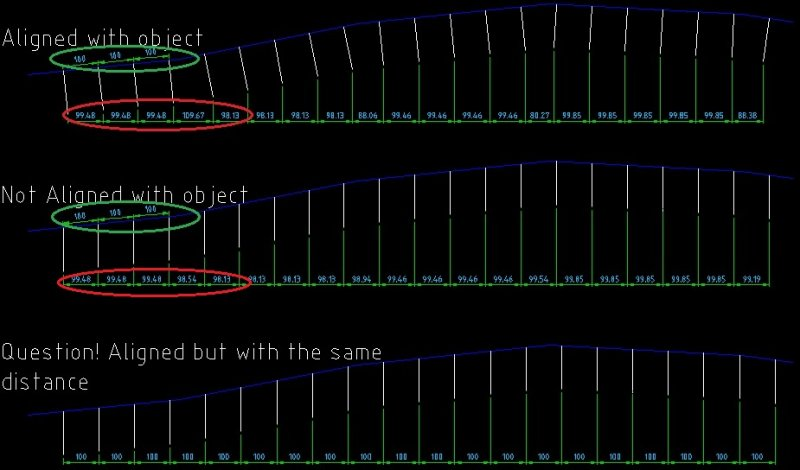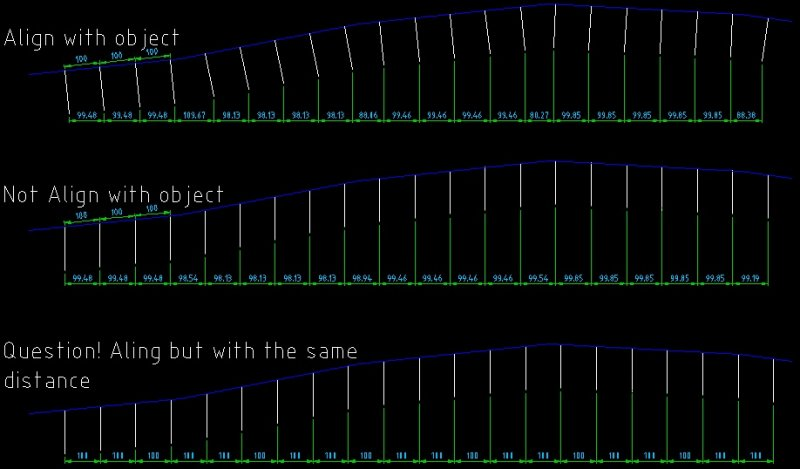Edited by CafeJr
##### Share on other sitesTry this quick hack:

```([color=BLUE]defun[/color] c:mymeasure ( [color=BLUE]/[/color] di en in ln ob p1 p2 sn sp x1 )
([color=BLUE]while[/color]
([color=BLUE]progn[/color]
([color=BLUE]setvar[/color] 'errno 0) ([color=BLUE]setq[/color] en ([color=BLUE]car[/color] ([color=BLUE]entsel[/color] [color=MAROON]"\nSelect object to measure: "[/color])))
([color=BLUE]cond[/color]
(   ([color=BLUE]=[/color] 7 ([color=BLUE]getvar[/color] 'errno))
([color=BLUE]princ[/color] [color=MAROON]"\nMissed, try again."[/color])
)
(   ([color=BLUE]=[/color] 'ename ([color=BLUE]type[/color] en))
([color=BLUE]if[/color] ([color=BLUE]vl-catch-all-error-p[/color] ([color=BLUE]vl-catch-all-apply[/color] '[color=BLUE]vlax-curve-getendparam[/color] ([color=BLUE]list[/color] en)))
([color=BLUE]princ[/color] [color=MAROON]"\nInvalid object selected."[/color])
)
)
)
)
)
([color=BLUE]if[/color] ([color=BLUE]and[/color] ([color=BLUE]=[/color] 'ename ([color=BLUE]type[/color] en))
([color=BLUE]progn[/color]
([color=BLUE]initget[/color] 6)
([color=BLUE]setq[/color] di ([color=BLUE]getdist[/color] [color=MAROON]"\nSpecify length of segment: "[/color]))
)
)
([color=BLUE]progn[/color]
([color=BLUE]setq[/color] p1 ([color=BLUE]vlax-curve-getstartpoint[/color] en)
p2 ([color=BLUE]vlax-curve-getendpoint[/color]   en)
x1 ([color=BLUE]abs[/color] ([color=BLUE]-[/color] ([color=BLUE]car[/color] p2) ([color=BLUE]car[/color] p1)))
sn ([color=BLUE]fix[/color] ([color=BLUE]/[/color] x1 di))
x1 ([color=BLUE]+[/color] ([color=BLUE]min[/color] ([color=BLUE]car[/color] p1) ([color=BLUE]car[/color] p2)) ([color=BLUE]/[/color] ([color=BLUE]-[/color] x1 ([color=BLUE]*[/color] di sn)) 2.0))
ob ([color=BLUE]vlax-ename->vla-object[/color] en)
([color=BLUE]if[/color] ([color=BLUE]=[/color] 1 ([color=BLUE]getvar[/color] 'cvport))
'paperspace
'modelspace
)
)
)
([color=BLUE]repeat[/color] ([color=BLUE]1+[/color] sn)
([color=BLUE]setq[/color] ln ([color=BLUE]vlax-invoke[/color] sp 'addline ([color=BLUE]list[/color] x1 0.0 0.0) ([color=BLUE]list[/color] x1 1.0 0.0)))
([color=BLUE]if[/color] ([color=BLUE]setq[/color] in ([color=BLUE]vlax-invoke[/color] ob 'intersectwith ln [color=BLUE]acextendotherentity[/color]))
([color=BLUE]entmake[/color] ([color=BLUE]list[/color] '(0 . [color=MAROON]"POINT"[/color]) ([color=BLUE]list[/color] 10 ([color=BLUE]car[/color] in) ([color=BLUE]cadr[/color] in) ([color=BLUE]caddr[/color] in))))
)
([color=BLUE]vla-delete[/color] ln)
([color=BLUE]setq[/color] x1 ([color=BLUE]+[/color] x1 di))
)
)
)
([color=BLUE]princ[/color])
)
```

##### Share on other sitesThis is a nice routine LEE, but how could we replace a 'BLOCKNAME' in lieu of your 'entmake POINT' ?

Thanks.

##### Share on other sites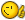Wowwwwwww... Thank you a lot "Lee Mac"!... It's exactly that I need!... I just need to exchange the point name to a block to be more fast on my application!... But it help me a lot!!!...
##### Share on other sitesYou're welcomeTry the following, change the highlighted block name to suit:

```([color=BLUE]defun[/color] c:mymeasure ( [color=BLUE]/[/color] *error* bd bn cm di en in ln ob p1 p2 sn sp x1 )

([color=BLUE]setq[/color] bn [color=MAROON][highlight]"myblock"[/highlight][/color]) [color=GREEN];; Name of block to insert[/color]

([color=BLUE]defun[/color] *error* ( msg )
([color=BLUE]if[/color] ([color=BLUE]and[/color] ([color=BLUE]=[/color] 'vla-object ([color=BLUE]type[/color] ln)) ([color=BLUE]not[/color] ([color=BLUE]vlax-erased-p[/color] ln)))
([color=BLUE]vl-catch-all-apply[/color] '[color=BLUE]vla-delete[/color] ([color=BLUE]list[/color] ln))
)
([color=BLUE]if[/color] ([color=BLUE]=[/color] 'int ([color=BLUE]type[/color] cm))
([color=BLUE]setvar[/color] 'cmdecho cm)
)
([color=BLUE]if[/color] ([color=BLUE]not[/color] ([color=BLUE]wcmatch[/color] ([color=BLUE]strcase[/color] msg [color=BLUE]t[/color]) [color=MAROON]"*break,*cancel*,*exit*"[/color]))
([color=BLUE]princ[/color] ([color=BLUE]strcat[/color] [color=MAROON]"\nError: "[/color] msg))
)
([color=BLUE]princ[/color])
)

([color=BLUE]cond[/color]
(   ([color=BLUE]=[/color] 4 ([color=BLUE]logand[/color] 4 ([color=BLUE]cdr[/color] ([color=BLUE]assoc[/color] 70 ([color=BLUE]tblsearch[/color] [color=MAROON]"layer"[/color] ([color=BLUE]getvar[/color] 'clayer))))))
([color=BLUE]princ[/color] [color=MAROON]"\nCurrent layer locked."[/color])
)
(   ([color=BLUE]not[/color]
([color=BLUE]or[/color] ([color=BLUE]tblsearch[/color] [color=MAROON]"block"[/color] bn)
([color=BLUE]and[/color] ([color=BLUE]setq[/color] bd ([color=BLUE]findfile[/color] ([color=BLUE]strcat[/color] bn [color=MAROON]".dwg"[/color])))
([color=BLUE]progn[/color]
([color=BLUE]setq[/color] cm ([color=BLUE]getvar[/color] 'cmdecho))
([color=BLUE]setvar[/color] 'cmdecho 0)
([color=BLUE]command[/color] [color=MAROON]"_.-insert"[/color] bd [color=BLUE]nil[/color])
([color=BLUE]setvar[/color] 'cmdecho cm)
([color=BLUE]tblsearch[/color] [color=MAROON]"block"[/color] bn)
)
)
)
)
)
(   ([color=BLUE]progn[/color]
([color=BLUE]while[/color]
([color=BLUE]progn[/color]
([color=BLUE]setvar[/color] 'errno 0) ([color=BLUE]setq[/color] en ([color=BLUE]car[/color] ([color=BLUE]entsel[/color] [color=MAROON]"\nSelect object to measure: "[/color])))
([color=BLUE]cond[/color]
(   ([color=BLUE]=[/color] 7 ([color=BLUE]getvar[/color] 'errno))
([color=BLUE]princ[/color] [color=MAROON]"\nMissed, try again."[/color])
)
(   ([color=BLUE]=[/color] 'ename ([color=BLUE]type[/color] en))
([color=BLUE]if[/color] ([color=BLUE]vl-catch-all-error-p[/color] ([color=BLUE]vl-catch-all-apply[/color] '[color=BLUE]vlax-curve-getendparam[/color] ([color=BLUE]list[/color] en)))
([color=BLUE]princ[/color] [color=MAROON]"\nInvalid object selected."[/color])
)
)
)
)
)
([color=BLUE]/=[/color] 'ename ([color=BLUE]type[/color] en))
)
)
(   ([color=BLUE]progn[/color]
([color=BLUE]initget[/color] 6)
([color=BLUE]setq[/color] di ([color=BLUE]getdist[/color] [color=MAROON]"\nSpecify length of segment: "[/color]))
)
([color=BLUE]setq[/color] p1 ([color=BLUE]vlax-curve-getstartpoint[/color] en)
p2 ([color=BLUE]vlax-curve-getendpoint[/color]   en)
x1 ([color=BLUE]abs[/color] ([color=BLUE]-[/color] ([color=BLUE]car[/color] p2) ([color=BLUE]car[/color] p1)))
sn ([color=BLUE]fix[/color] ([color=BLUE]/[/color] x1 di))
x1 ([color=BLUE]+[/color] ([color=BLUE]min[/color] ([color=BLUE]car[/color] p1) ([color=BLUE]car[/color] p2)) ([color=BLUE]/[/color] ([color=BLUE]-[/color] x1 ([color=BLUE]*[/color] di sn)) 2.0))
ob ([color=BLUE]vlax-ename->vla-object[/color] en)
([color=BLUE]if[/color] ([color=BLUE]=[/color] 1 ([color=BLUE]getvar[/color] 'cvport))
'paperspace
'modelspace
)
)
)
([color=BLUE]repeat[/color] ([color=BLUE]1+[/color] sn)
([color=BLUE]setq[/color] ln ([color=BLUE]vlax-invoke[/color] sp 'addline ([color=BLUE]list[/color] x1 0.0 0.0) ([color=BLUE]list[/color] x1 1.0 0.0)))
([color=BLUE]if[/color] ([color=BLUE]setq[/color] in ([color=BLUE]vlax-invoke[/color] ob 'intersectwith ln [color=BLUE]acextendotherentity[/color]))
([color=BLUE]vlax-invoke[/color] sp 'insertblock ([color=BLUE]mapcar[/color] '[color=BLUE]+[/color] in '(0.0 0.0 0.0)) bn 1.0 1.0 1.0 0.0)
)
([color=BLUE]vla-delete[/color] ln)
([color=BLUE]setq[/color] x1 ([color=BLUE]+[/color] x1 di))
)
)
)
([color=BLUE]princ[/color])
)
```

##### Share on other sites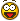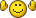"Lee Mac"!... It works good!... Thanks a lot!!!...
##### Share on other sitesI put on code a litle question to got the Block Name:

```(defun c:mymeasure ( / *error* bd bn cm di en in ln ob p1 p2 sn sp x1 )
;(setq bn "Botão") ; Name of block to insert ("myblock")
[color=red](setq bn (getstring "\nEnter with block Name: "))[/color]
(defun *error* ( msg )

(if (and (= 'vla-object (type ln)) (not (vlax-erased-p ln)))
(vl-catch-all-apply 'vla-delete (list ln))
)
(if (= 'int (type cm))
(setvar 'cmdecho cm)
)
(if (not (wcmatch (strcase msg t) "*break,*cancel*,*exit*"))
(princ (strcat "\nError: " msg))
)
(princ)
)
(cond
(   (= 4 (logand 4 (cdr (assoc 70 (tblsearch "layer" (getvar 'clayer))))))
(princ "\nCurrent layer locked.")
)
(   (not
(or (tblsearch "block" bn)
(and (setq bd (findfile (strcat bn ".dwg")))
(progn
(setq cm (getvar 'cmdecho))
(setvar 'cmdecho 0)
(command "_.-insert" bd nil)
(setvar 'cmdecho cm)
(tblsearch "block" bn)
)
)
)
)
)
(   (progn
(while
(progn
(setvar 'errno 0) (setq en (car (entsel "\nSelect object to measure: ")))
(cond
(   (= 7 (getvar 'errno))
(princ "\nMissed, try again.")
)
(   (= 'ename (type en))
(if (vl-catch-all-error-p (vl-catch-all-apply 'vlax-curve-getendparam (list en)))
(princ "\nInvalid object selected.")
)
)
)
)
)
(/= 'ename (type en))
)
)
(   (progn
(initget 6)
(setq di (getdist "\nSpecify length of segment: "))
)
(setq p1 (vlax-curve-getstartpoint en)
p2 (vlax-curve-getendpoint   en)
x1 (abs (- (car p2) (car p1)))
sn (fix (/ x1 di))
x1 (+ (min (car p1) (car p2)) (/ (- x1 (* di sn)) 2.0))
ob (vlax-ename->vla-object en)
(if (= 1 (getvar 'cvport))
'paperspace
'modelspace
)
)
)
(repeat (1+ sn)
(setq ln (vlax-invoke sp 'addline (list x1 0.0 0.0) (list x1 1.0 0.0)))
(if (setq in (vlax-invoke ob 'intersectwith ln acextendotherentity))
(vlax-invoke sp 'insertblock (mapcar '+ in '(0.0 0.0 0.0)) bn 1.0 1.0 1.0 0.0)
)
(vla-delete ln)
(setq x1 (+ x1 di))
)
)
)
(princ)
)

##### Share on other sitesYou're welcome!## Join the conversation

You can post now and register later. If you have an account, sign in now to post with your account.
Note: Your post will require moderator approval before it will be visible.×   Pasted as rich text.   Restore formatting

Only 75 emoji are allowed.